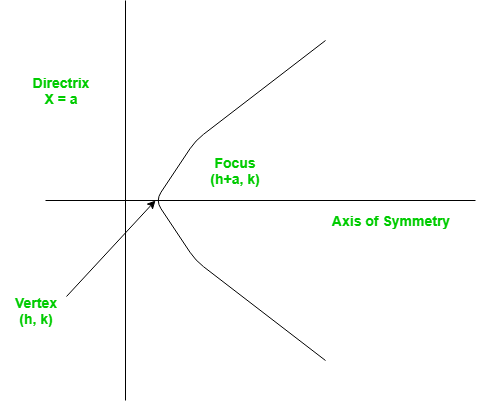Open In App

# Python Program for Finding the vertex, focus and directrix of a parabola

A set of points on a plain surface that forms a curve such that any point on that curve is equidistant from the focus is a parabola.
Vertex of a parabola is the coordinate from which it takes the sharpest turn whereas a is the straight line used to generate the curve.The standard form of a parabola equation is. Given the values of a, b and c; our task is to find the coordinates of vertex, focus and the equation of the directrix.

Example –

```Input : 5 3 2
Output : Vertex:(-0.3, 1.55)
Focus: (-0.3, 1.6)
Directrix: y=-198
Consult the formula below for explanation.
```